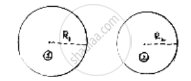Share

# The Sum of the Radii of Two Circles is 140 Cm and the Difference of Their Circumferences in 88 Cm. Find the Diameters of the Circles. - CBSE Class 10 - Mathematics

ConceptPerimeter and Area of a Circle

#### Question

The sum of the radii of two circles is 140 cm and the difference of their circumferences in 88 cm. Find the diameters of the circles.

#### SolutionLet radius of circles be 𝑟1𝑎𝑛𝑑 𝑟2

Given sum of radius = 140cm

𝑟1 + 𝑟2 = 140 …..(i)

difference in circumferences = 88 𝑐𝑚

2 × 𝑟1 − 2𝜋𝑟2 = 88

2 × 22/7 (r_1 − r_2) 88

r_1-r_2 = (88xx7)/(2xx22) = 14

r_1 = r_2 +14….(ii)

(𝑖𝑖)𝑖𝑛 (𝑖) ⇒ r_1 + r_2 +14 =140

⇒ 2𝑟2 = 126

⇒ 𝑟2 = 126/2 = 63𝑐𝑚𝑠

𝑟2 = 63𝑐𝑚𝑠 𝑖𝑛 (𝑖𝑖) 𝑟1 = 63 + 14 = 77 𝑐𝑚𝑠

Diameter of circle (i) = 2𝑟1 = 2 × 77 = 154 𝑐𝑚𝑠

Diameter of circle (ii) = 2𝑟2 = 2 × 63 = 126 𝑐𝑚𝑠

Is there an error in this question or solution?

#### Video TutorialsVIEW ALL 

Solution The Sum of the Radii of Two Circles is 140 Cm and the Difference of Their Circumferences in 88 Cm. Find the Diameters of the Circles. Concept: Perimeter and Area of a Circle.
S